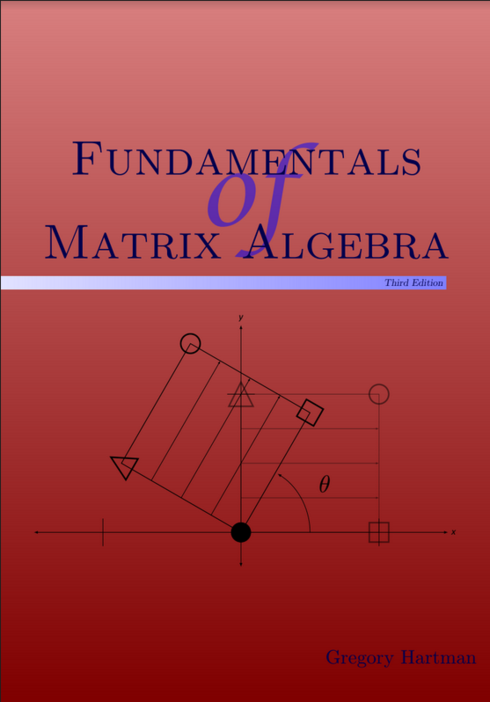# Fundamentals of Matrix Algebra

(1 review)Gregory Hartman, Virginia Military Institute

Publisher: APEX Calculus

Language: English

## Conditions of UseAttribution-NonCommercial
CC BY-NC

## Reviews

1 Systems of Linear Equations

• 1.1 Introduction to Linear Equations
• 1.2 Using Matrices To Solve Systems of Linear Equations
• 1.3 Elementary Row Operations and Gaussian Elimination
• 1.4 Existence and Uniqueness of Solutions
• 1.5 Applications of Linear Systems

2 Matrix Arithmetic

• 2.1 Matrix Addition and Scalar Multiplication
• 2.2 Matrix Multiplication
• 2.3 Visualizing Matrix Arithmetic in 2D
• 2.4 Vector Solutions to Linear Systems
• 2.5 Solving Matrix Equations AX = B
• 2.6 The Matrix Inverse
• 2.7 Properties of the Matrix Inverse

3 Operations on Matrices

• 3.1 The Matrix Transpose
• 3.2 The Matrix Trace
• 3.3 The Determinant
• 3.4 Properties of the Determinant
• 3.5 Cramer’s Rule

4 Eigenvalues and Eigenvectors

• 4.1 Eigenvalues and Eigenvectors
• 4.2 Properties of Eigenvalues and Eigenvectors

5 Graphical Explorations of Vectors

• 5.1 Transformations of the Cartesian Plane
• 5.2 Properties of Linear Transformations
• 5.3 Visualizing Vectors: Vectors in Three Dimensions

## Ancillary Material

• Submit ancillary resource